# How not to screw up your units

Units of values are very important, but also easy to screw up. The F# programming language found a nice solution to this problem. Because units are all over the place in an average PLC project, I started to think about how to replicate this behavior in TwinCAT. Eventually I found a method which gives compiler errors if the wrong units are used.

Back when I had my first science classes, the teachers would always emphasize the importance of units. If I forgot them, points were usually subtracted from my final mark. At the time I always found this a bit unreasonable. I was calculating a speed, of course the units would be in km/h!

Over the years I got wiser and started to get the importance of what units are used. I learned that things can go horribly wrong when this is not done. For example, the Mars Climate Orbiter failed to go into orbit around the red planet, because of a mix-up between metric and imperial units.

Now, most of us are not working with hundred million dollar machines which try to orbit a distant planet. But also for less expensive machines on earth it would be nice to not make these mistakes. Below I’ll show the different options I thought of how to prevent unit mix-ups. They are ranked from worst to best, according to my opinion.

## Unit checks in F#

Before diving into how unit checks can be done in TwinCAT, let me first show you how unit checks are done in F#. In F# a unit can be defined using the following syntax.

``````[<Measure>] type Celsius
[<Measure>] type Fahrenheit
``````

These units can be used in functions. For example, below a function is defined with `let` called `CelsiusToFahrenheit`. It has a single input argument called `temperature`. Inside the function the conversion takes place using the units defined above. The units are placed between angle brackets `<` and `>`.

``````let CelsiusToFahrenheit temperature =
temperature * 1.8<Fahrenheit/Celsius> + 32.0<Fahrenheit>
``````

The unit of `temperature` does not need to be supplied, as these will be inferred by the compiler from the units of the variables inside. Now, if we would like to use this function, we also have to use the correct input units.

``````> CelsiusToFahrenheit 21.0<Celsius>;;
val it : float<Fahrenheit> = 69.8
``````

If we use the wrong units the compiler will complain.

``````> CelsiusToFahrenheit 62.0<Fahrenheit>;;

CelsiusToFahrenheit 62.0<Fahrenheit>;;
--------------------^^^^^^^^^^^^^^^^

stdin(30,21): error FS0001: Type mismatch. Expecting a
'float<Celsius>'
but given a
'float<Fahrenheit>'
The unit of measure 'Celsius' does not match the unit of measure 'Fahrenheit'
``````

Likewise, if the unit is omitted we also get a compiler error.

``````> CelsiusToFahrenheit 94.0;;

CelsiusToFahrenheit 94.0;;
--------------------^^^^

stdin(32,21): error FS0001: This expression was expected to have type
'float<Celsius>'
but here has type
'float'
``````

I think this is a very useful feature. If you want to learn more about it, you can read an excellent overview in F# for Fun and Profit. Now let’s explore how this functionality can be implemented in TwinCAT!

## 4. Putting units in comments

Probably the most commonly used method is to put the units of a variable in the comment. For example

``````maximumPressure : REAL; // [mbar]
``````

However, it is very hard to spot a mistake as shown by the following example.

``````PROGRAM MAIN
VAR
pressure1 : REAL; // [mbar]
pressure2 : REAL; // [Torr]
END_VAR

pressure2 := TorrToMbar(pressure1);
``````

The pros and cons of this method are:

✔️ There is a unit.

❌ Need to hover over it to see the unit.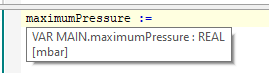❌ No automatic checks to ensure the right unit is used.

## 3. Using aliases

Aliases can be used to define a unit. An alias is just another name for a standard data type. Some standard TwinCAT aliases you might have seen are `T_MaxString` = `STRING(255)` or `FLOAT` = `REAL`.

An alias can be created by right clicking on the PLC project and choose Add > DUT. Then in the following screen select Alias and give it a name (e.g. mbar) and a datatype (e.g. `REAL`).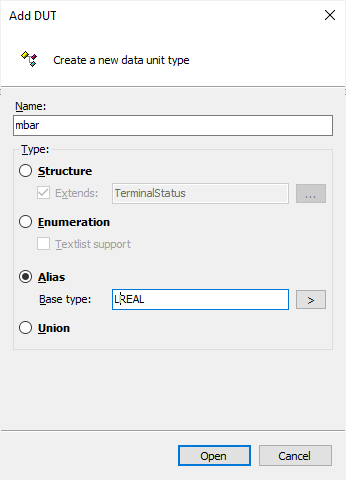Then it can be used as follows.

``````PROGRAM MAIN
VAR
pressure_si : mbar;
pressure_imperial : Torr;
END_VAR

pressure_si := TorrToMbarWithAlias(pressure:=pressure_imperial);
``````

where `TorrToMbarWithAlias` is defined as

``````FUNCTION TorrToMbarWithAlias : mbar
VAR_INPUT
pressure : Torr;
END_VAR

TorrToMbarWithAlias := pressure * 1.3332236842;
``````

At the first glance this looks very similar to the F# approach. However, the aliases are just a different name for the underlying datatype. The compiler only checks that the underlying datatype (`REAL` in this case), is correct. So the following would be possible:

``````pressure_in_torr := TorrToMbarWithAlias(pressure:=pressure_in_mbar);
``````

Concluding

✔️ There is a unit.

❌ Need to hover over it to see the unit.

❌ Compiler doesn’t complain if the wrong alias is used.

## 2. Add unit to the variable name

Another option would be to append the unit to the variable name:

``````maximumPressure_mbar : REAL;
``````

This method also doesn’t have any automatic check to prevent unit mix-ups. But the following mistake is harder to make, because the input variable’s unit doesn’t match the argument name one.

``````PROGRAM MAIN
VAR
pressure1_mbar : REAL;
pressure2_Torr : REAL;
END_VAR

pressure2_Torr := TorrToMbarWithUnits(pressure_Torr:=pressure1_mbar);
``````

✔️ There is a unit.

✔️ No need to hover over it to see the unit.

❌ No automatic checks to ensure the right unit is used.

## 1. Using structs

We’re making progress, but the compiler is not helping us yet. In order for the compiler to help us, STRUCTs can be used. You can add a STRUCT by right clicking on your PLC project and selecting Add > DUT.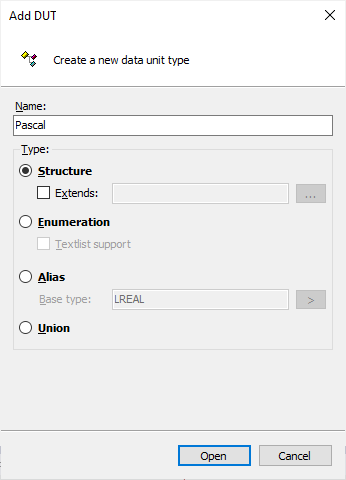And then define the STRUCTs as follows.

``````TYPE Pascal :
STRUCT
_ : REAL;
END_STRUCT
END_TYPE
``````
``````TYPE PoundsPerSquareInch :
STRUCT
_ : REAL;
END_STRUCT
END_TYPE
``````

We then add a conversion function.

``````FUNCTION PoundsPerSquareInchToPascal : Pascal
VAR_INPUT
pressure : PoundsPerSquareInch;
END_VAR

PoundsPerSquareInchToPascal._ := pressure._ * 6895;
``````

If we try to run this function with the wrong units:

``````PROGRAM MAIN
VAR
pressure_eu : Pascal;
pressure_us : PoundsPerSquareInch;
END_VAR

pressure_us := PoundsPerSquareInchToPascal(pressure:=pressure_eu);
``````

the compiler will complain. 🙌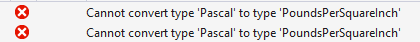✔️ There is a unit.

❌ Need to hover over it to see the unit, but this is a minor issue since the compiler will check it for us.

✔️ Compiler complains if the wrong type is used.

❌ The interface is not very convenient, since we have to append `._` each time we want to access the value.

Using structs gives us a major advantage since the compiler will help us. However, it feels a bit excessive to define a whole struct for this and then we have to use the `._` to assign or use the variable inside. We can ease the pain a bit by adding some useful information to the struct. For example, we could add the units to the struct in string format. This string could then be used in the HMI for example.

``````TYPE mmHg :
STRUCT
_ : REAL;
unit : STRING := 'mmHg';
END_STRUCT
END_TYPE
``````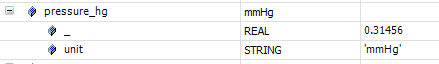Another option could be to add some information about the state of the input signal. Terminals usually have a WcState variable. This variable shows if the terminal cyclically exchanges data with the master and does this without errors. Furthermore, some input channels also have a state, which can show if there is an open circuit, short circuit or some other irregularity.

To capture the terminal status we make a new struct.

``````TYPE TerminalStatus :
STRUCT
WorkingCounterError : BOOL;
IOError : BOOL;
END_STRUCT
END_TYPE
``````

Then whenever we define a new unit, we extend the struct above.

``````TYPE Celsius EXTENDS TerminalStatus :
STRUCT
_ : REAL;
END_STRUCT
END_TYPE
``````

In order to check whether a signal is valid, we can define a new function. The function uses the general `TerminalStatus` as its input type. That way this function can be called with extended structs, such as `Celsius`, as I wrote about in an earlier article.

``````FUNCTION IsValid : BOOL
VAR_INPUT
status : TerminalStatus;
END_VAR

IsValid := NOT (status.IOError OR status.WorkingCounterError);
``````

Finally we define a conversion function, where we only convert the temperature if the signal state is ok.

``````FUNCTION FahrenheitToCelsius : Celsius
VAR_INPUT
temperature : Fahrenheit;
END_VAR

FahrenheitToCelsius.IOError := temperature.IOError;
FahrenheitToCelsius.WorkingCounterError := temperature.WorkingCounterError;
IF IsValid(temperature) THEN
FahrenheitToCelsius._ := (temperature._ - 32) /  1.8;
END_IF
``````

After activating this code we can see it in action.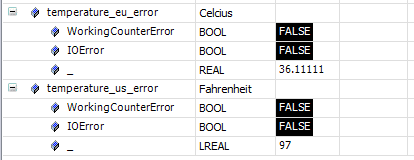## Conclusions

In the end we came quite close to the F# unit checks implementation. Although the intermediate conversions, such as the 32 in `FahrenheitToCelsius`, are not checked by the compiler as in F#. But these intermediate conversions can be checked relatively easy with unit tests. What is checked with the method above are the in and outputs of the function. I would argue this is the most important part, since they usually need integration tests. Finally the interface of a custom struct is not the most convenient, but I showed some examples how you could soften the pain by adding some useful information to the unit structs.

Like my work? Consider a donation!